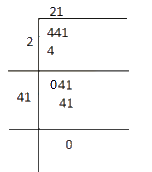# Ex.6.4 Q6 Squares and Square Roots - NCERT Maths Class 8

Go back to  'Ex.6.4'

## Question

Find the length of the side of a square whose area is $$441\;\rm{m^2}$$ .

Video Solution
Squares And Square Roots
Ex 6.4 | Question 6

## Text Solution

What is known?

Area of the square

What is unknown?

Length of the side of a square

Reasoning:

Since, area of a square is equals to square of its side.

The length of the side can be calculated by finding the square root of the area.

Steps:

Area of the square = side of a square x side of a square

\begin{align}441\;\rm{m^2} = \text{(side of a square)}^2\end{align}$${\text{Side of a square}} = \;\sqrt {441}= 21\;{\rm{m}}$$

Learn from the best math teachers and top your exams

• Live one on one classroom and doubt clearing
• Practice worksheets in and after class for conceptual clarity
• Personalized curriculum to keep up with school